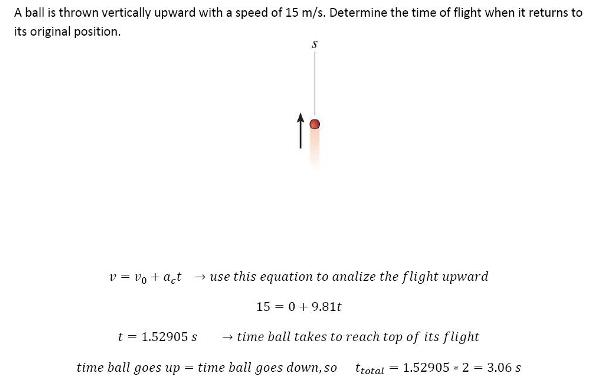dynamics math problems engineering equations dynamics basic mechanics school homework engineering math dynamics formulas dynamics problems dynamics problem solutions to dynamics problems full solution dynamics engineering dynamics problem solution dynamics math problems engineering equations dynamics basic mechanics school homework engineering math dynamics formulas dynamics problems dynamics problem solutions to dynamics problems full solution dynamics engineering dynamics problem solution
dynamics math problems engineering equations dynamics basic mechanics school homework engineering math dynamics formulas dynamics problems dynamics problem solutions to dynamics problems full solution dynamics engineering dynamics problem solution dynamics math problems engineering equations dynamics basic mechanics school homework engineering math dynamics formulas dynamics problems dynamics problem solutions to dynamics problems full solution dynamics engineering dynamics problem solution